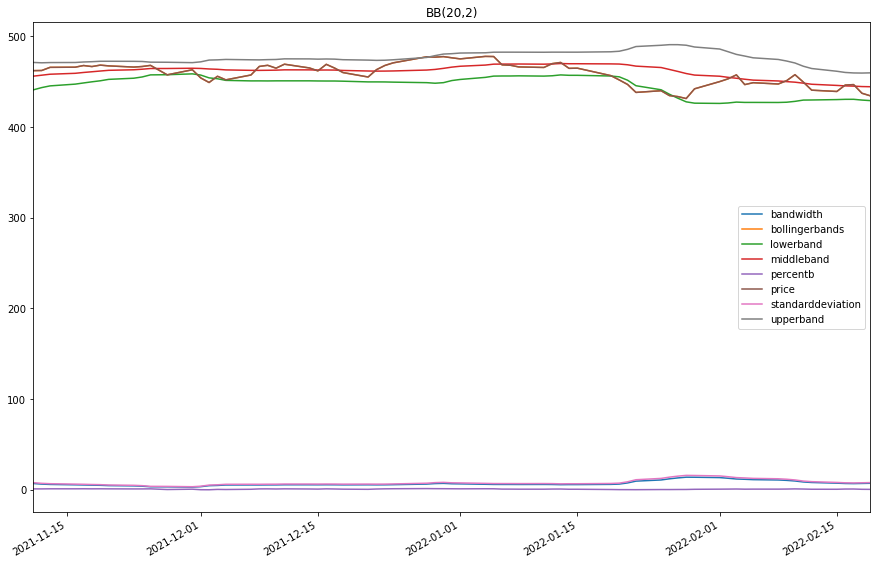# Indicator Reference

## Bollinger Bands

### Introduction

This indicator creates a moving average (middle band) with an upper band and lower band fixed at k standard deviations above and below the moving average.

### Create Manual Indicators

You can manually create a BollingerBands indicator, so it doesn’t automatically update. Manual indicators let you update their values with any data you choose. The following reference table describes the BollingerBands constructor.

### BollingerBands()1/2

            BollingerBands QuantConnect.Indicators.BollingerBands (
int                 period,
decimal             k,
*MovingAverageType  movingAverageType
)


Initializes a new instance of the BollingerBands class.

### BollingerBands()2/2

            BollingerBands QuantConnect.Indicators.BollingerBands (
string              name,
int                 period,
decimal             k,
*MovingAverageType  movingAverageType
)


Initializes a new instance of the BollingerBands class.

The following table shows the MovingAverageType enumeration members and the indicator that implements each one:

MovingAverageTypeUnderlying Indicator
SimpleSimple Moving Average
ExponentialExponential Moving Average
WildersWilder Moving Average
LinearWeightedMovingAverageLinear Weighted Moving Average
DoubleExponentialDouble Exponential Moving Average
TripleExponentialTriple Exponential Moving Average
TriangularTriangular Moving Average
T3T3 Moving Average
KamaKaufman Adaptive Moving Average
HullHull Moving Average
AlmaArnaud Legoux Moving Average

### Update Manual Indicators

You can update the indicator automatically or manually.

#### Automatic Update

To register a manual indicator for automatic updates with the security data, call the RegisterIndicator method.


private BollingerBands _bb;

// In Initialize()
_bb = new BollingerBands(name, period, k);
_bb.Updated += IndicatorUpdateMethod;

RegisterIndicator(symbol, _bb, Resolution.Daily);

// In IndicatorUpdateMethod()
{
var indicatorValue = _bb.Current.Value;
}

# In Initialize()
self.bb = BollingerBands(name, period, k)
self.bb.Updated += self.IndicatorUpdateMethod

self.RegisterIndicator(symbol, self.bb, Resolution.Daily)

# In IndicatorUpdateMethod()
indicator_value = self.bb.Current.Value

To customize the data that automatically updates the indicator, see Custom Indicator Periods and Custom Indicator Values.

#### Manual Update

Updating your indicator manually enables you to control when the indicator is updated and what data you use to update it. To manually update the indicator, call the Update method with time/decimal pair. The indicator will only be ready after you prime it with enough data.


private BollingerBands _bb;
private Symbol symbol;

// In Initialize()
_bb = new BollingerBands(period, k);

// In OnData()
if (data.ContainsKey(_symbol))
{
_bb.Update(data[symbol].EndTime, data[symbol].High);
}
{
var indicatorValue = _bb.Current.Value;
}

# In Initialize()
self.bb = BollingerBands(period, k)

# In OnData()
if data.ContainsKey(self.symbol):
self.bb.Update(data[self.symbol].EndTime, data[self.symbol].High)
indicator_value = self.bb.Current.Value

### Create Automatic Indicators

The BB method creates an BollingerBands indicator, sets up a consolidator to update the indicator, and then returns the indicator so you can use it in your algorithm.

The following reference table describes the BB method:

### BB()1/1

            BollingerBands QuantConnect.Algorithm.QCAlgorithm.BB (
Symbol                           symbol,
Int32                            period,
Decimal                          k,
*MovingAverageType               movingAverageType,
*Nullable<Resolution>      resolution,
*Func<IBaseData, Decimal>  selector
)


Creates a new BollingerBands indicator which will compute the MiddleBand, UpperBand, LowerBand, and StandardDeviation

The following table shows the MovingAverageType enumeration members and the indicator that implements each one:

MovingAverageTypeUnderlying Indicator
SimpleSimple Moving Average
ExponentialExponential Moving Average
WildersWilder Moving Average
LinearWeightedMovingAverageLinear Weighted Moving Average
DoubleExponentialDouble Exponential Moving Average
TripleExponentialTriple Exponential Moving Average
TriangularTriangular Moving Average
T3T3 Moving Average
KamaKaufman Adaptive Moving Average
HullHull Moving Average
AlmaArnaud Legoux Moving Average

If you don't provide a resolution, it defauls to the security resolution. If you provide a resolution, it must be greater than or equal to the resolution of the security. For instance, if you subscribe to hourly data for a security, you should update its indicator with data that spans 1 hour or longer.

### Get Indicator Values

To get the value of the indicator, use its Current.Value attribute.

private BollingerBands _bb;

// In Initialize()
_bb = BB(symbol, period, k);

// In OnData()
{
var standardDeviation = _bb.StandardDeviation.Current.Value;
var middleBand = _bb.MiddleBand.Current.Value;
var upperBand = _bb.UpperBand.Current.Value;
var lowerBand = _bb.LowerBand.Current.Value;
var bandWidth = _bb.BandWidth.Current.Value;
var percentB = _bb.PercentB.Current.Value;
var price = _bb.Price.Current.Value;
var current = _bb.Current.Value;
}
# In Initialize()
self.bb = self.BB(symbol, period, k)

# In OnData()
standard_deviation = self.bb.StandardDeviation.Current.Value
middle_band = self.bb.MiddleBand.Current.Value
upper_band = self.bb.UpperBand.Current.Value
lower_band = self.bb.LowerBand.Current.Value
band_width = self.bb.BandWidth.Current.Value
percent_b = self.bb.PercentB.Current.Value
price = self.bb.Price.Current.Value
current = self.bb.Current.Value


### Visualization

To plot indicator values, in the OnData event handler, call the Plot method.

private BollingerBands _bb;

// In Initialize()
_bb = BB(symbol, period, k);

// In OnData()
{
Plot("My Indicators", "standarddeviation", _bb.StandardDeviation);
Plot("My Indicators", "middleband", _bb.MiddleBand);
Plot("My Indicators", "upperband", _bb.UpperBand);
Plot("My Indicators", "lowerband", _bb.LowerBand);
Plot("My Indicators", "bandwidth", _bb.BandWidth);
Plot("My Indicators", "percentb", _bb.PercentB);
Plot("My Indicators", "price", _bb.Price);
Plot("My Indicators", "bollingerbands", _bb.Current);
}
# In Initialize()
self.bb = self.BB(symbol, period, k)

# In OnData()
self.Plot("My Indicators", "standarddeviation", self.bb.StandardDeviation)
self.Plot("My Indicators", "middleband", self.bb.MiddleBand)
self.Plot("My Indicators", "upperband", self.bb.UpperBand)
self.Plot("My Indicators", "lowerband", self.bb.LowerBand)
self.Plot("My Indicators", "bandwidth", self.bb.BandWidth)
self.Plot("My Indicators", "percentb", self.bb.PercentB)
self.Plot("My Indicators", "price", self.bb.Price)
self.Plot("My Indicators", "bollingerbands", self.bb.Current)